# 7884 Results

View
Selected filters:
• Upper ElementaryUnrestricted Use
CC BY
Rating

This is a task from the Illustrative Mathematics website that is one part of a complete illustration of the standard to which it is aligned. Each task has at least one solution and some commentary that addresses important aspects of the task and its potential use.

Subject:
Mathematics
Material Type:
Activity/Lab
Provider:
Illustrative Mathematics
Provider Set:
Illustrative Mathematics
Author:
Illustrative Mathematics
07/02/2018Unrestricted Use
CC BY
Rating

This is a task from the Illustrative Mathematics website that is one part of a complete illustration of the standard to which it is aligned. Each task has at least one solution and some commentary that addresses important aspects of the task and its potential use.

Subject:
Mathematics
Material Type:
Activity/Lab
Provider:
Illustrative Mathematics
Provider Set:
Illustrative Mathematics
Author:
Illustrative Mathematics
07/02/2018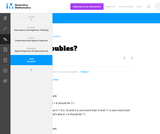Unrestricted Use
CC BY
Rating

This is a task from the Illustrative Mathematics website that is one part of a complete illustration of the standard to which it is aligned. Each task has at least one solution and some commentary that addresses important aspects of the task and its potential use.

Subject:
Mathematics
Material Type:
Activity/Lab
Provider:
Illustrative Mathematics
Provider Set:
Illustrative Mathematics
Author:
Illustrative Mathematics
07/02/2018Unrestricted Use
CC BY
Rating

This is a task from the Illustrative Mathematics website that is one part of a complete illustration of the standard to which it is aligned. Each task has at least one solution and some commentary that addresses important aspects of the task and its potential use.

Subject:
Mathematics
Material Type:
Activity/Lab
Provider:
Illustrative Mathematics
Provider Set:
Illustrative Mathematics
Author:
Illustrative Mathematics
07/02/2018Unrestricted Use
CC BY
Rating

This is a task from the Illustrative Mathematics website that is one part of a complete illustration of the standard to which it is aligned. Each task has at least one solution and some commentary that addresses important aspects of the task and its potential use.

Subject:
Mathematics
Material Type:
Activity/Lab
Provider:
Illustrative Mathematics
Provider Set:
Illustrative Mathematics
Author:
Illustrative Mathematics
07/02/2018Conditional Remix & Share Permitted
CC BY-NC-SA
Rating

SFUSD's creative computing curriculum introduces computer science as a creative, collaborative, and engaging discipline to children in third through fifth grade.

Across 15-20 lessons at each grade level, students will learn about algorithms and programming, computing systems, the Internet, and impacts of computing, while developing strong practices and dispositions. Lessons are designed to be implemented in 45 to 60-minute periods approximately once per week.

Subject:
Information Technology Education
Educational Technology
Computer Science
Material Type:
Activity/Lab
Full Course
Lesson
Author:
Adapted primarily from Creative Commons licensed resources developed by the ScratchEd team at the Harvard Graduate School of Education and Code.org. See also the original ScratchEd Creative Computing curriculum guide.
Created by the San Francisco Unified School District (SFUSD) Computer Science team: CSinSF.org.
03/21/2019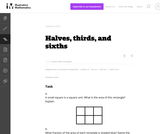Unrestricted Use
CC BY
Rating

This is a task from the Illustrative Mathematics website that is one part of a complete illustration of the standard to which it is aligned. Each task has at least one solution and some commentary that addresses important asects of the task and its potential use. Here are the first few lines of the commentary for this task: A small square is a square unit. What is the area of this rectangle? Explain. What fraction of the area of each rectangle is shaded blue? Name the frac...

Subject:
Elementary Mathematics
Material Type:
Activity/Lab
Provider:
Illustrative Mathematics
Provider Set:
Illustrative Mathematics
Author:
Illustrative Mathematics
05/16/2013Unrestricted Use
CC BY
Rating

This is a task from the Illustrative Mathematics website that is one part of a complete illustration of the standard to which it is aligned. Each task has at least one solution and some commentary that addresses important asects of the task and its potential use. Here are the first few lines of the commentary for this task: Find the area of each colored figure. Each grid square is 1 inch long....

Subject:
Elementary Mathematics
Material Type:
Activity/Lab
Provider:
Illustrative Mathematics
Provider Set:
Illustrative Mathematics
Author:
Illustrative Mathematics
05/27/2013Unrestricted Use
CC BY
Rating

This is a task from the Illustrative Mathematics website that is one part of a complete illustration of the standard to which it is aligned. Each task has at least one solution and some commentary that addresses important aspects of the task and its potential use.

Subject:
Mathematics
Material Type:
Activity/Lab
Provider:
Illustrative Mathematics
Provider Set:
Illustrative Mathematics
Author:
Illustrative Mathematics
07/02/2018Unrestricted Use
CC BY
Rating

This is a task from the Illustrative Mathematics website that is one part of a complete illustration of the standard to which it is aligned. Each task has at least one solution and some commentary that addresses important aspects of the task and its potential use.

Subject:
Mathematics
Material Type:
Activity/Lab
Provider:
Illustrative Mathematics
Provider Set:
Illustrative Mathematics
Author:
Illustrative Mathematics
07/02/2018Unrestricted Use
CC BY
Rating

This is a task from the Illustrative Mathematics website that is one part of a complete illustration of the standard to which it is aligned. Each task has at least one solution and some commentary that addresses important aspects of the task and its potential use.

Subject:
Mathematics
Material Type:
Activity/Lab
Provider:
Illustrative Mathematics
Provider Set:
Illustrative Mathematics
Author:
Illustrative Mathematics
07/02/2018Unrestricted Use
CC BY
Rating

This is a task from the Illustrative Mathematics website that is one part of a complete illustration of the standard to which it is aligned. Each task has at least one solution and some commentary that addresses important asects of the task and its potential use. Here are the first few lines of the commentary for this task: Draw a purple pentagon Draw a blue shape with 3 line segments that is not a triangle. Draw an orange shape with 4 line segments that is not a quadrilat...

Subject:
Elementary Mathematics
Material Type:
Activity/Lab
Provider:
Illustrative Mathematics
Provider Set:
Illustrative Mathematics
Author:
Illustrative Mathematics
05/27/2013Unrestricted Use
CC BY
Rating

This is a task from the Illustrative Mathematics website that is one part of a complete illustration of the standard to which it is aligned. Each task has at least one solution and some commentary that addresses important asects of the task and its potential use. Here are the first few lines of the commentary for this task: Plot the following numbers on the number line: 80 328 791 1. Round each number to the nearest 100. How can you see this on the number line? 2. Round ea...

Subject:
Elementary Mathematics
Material Type:
Activity/Lab
Provider:
Illustrative Mathematics
Provider Set:
Illustrative Mathematics
Author:
Illustrative Mathematics
02/18/2014Unrestricted Use
CC BY
Rating

This is a task from the Illustrative Mathematics website that is one part of a complete illustration of the standard to which it is aligned. Each task has at least one solution and some commentary that addresses important asects of the task and its potential use. Here are the first few lines of the commentary for this task: There are 6 tables in Mrs. Potter's art classroom. There are 4 students sitting at each table. Each student has a box of 10 colored pencils. (A) How ma...

Subject:
Elementary Mathematics
Material Type:
Activity/Lab
Provider:
Illustrative Mathematics
Provider Set:
Illustrative Mathematics
Author:
Illustrative Mathematics
05/17/2013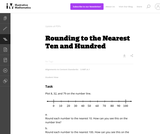Unrestricted Use
CC BY
Rating

This is a task from the Illustrative Mathematics website that is one part of a complete illustration of the standard to which it is aligned. Each task has at least one solution and some commentary that addresses important asects of the task and its potential use. Here are the first few lines of the commentary for this task: Plot 8, 32, and 79 on the number line. 1. Round each number to the nearest 10. How can you see this on the number line? 2. Round each number to the nea...

Subject:
Elementary Mathematics
Material Type:
Activity/Lab
Provider:
Illustrative Mathematics
Provider Set:
Illustrative Mathematics
Author:
Illustrative Mathematics
02/18/2014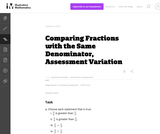Unrestricted Use
CC BY
Rating

This is a task from the Illustrative Mathematics website that is one part of a complete illustration of the standard to which it is aligned. Each task has at least one solution and some commentary that addresses important asects of the task and its potential use. Here are the first few lines of the commentary for this task: Choose each statement that is true. $\frac34$ is greater than $\frac54$. $\frac54$ is greater than $\frac34$. $\frac34 \gt \frac54$. \frac34 \lt \frac... Subject: Elementary Mathematics Material Type: Activity/Lab Provider: Illustrative Mathematics Provider Set: Illustrative Mathematics Author: Illustrative Mathematics Date Added: 03/18/2013Unrestricted Use CC BY Rating This is a task from the Illustrative Mathematics website that is one part of a complete illustration of the standard to which it is aligned. Each task has at least one solution and some commentary that addresses important asects of the task and its potential use. Here are the first few lines of the commentary for this task: Choose each statement that is true.\frac98$is greater than$\frac{9}{4}$.$\frac{9}{4}$is greater than$\frac98$.$\frac98 \gt \frac{9}{4}$.$\frac...

Subject:
Elementary Mathematics
Material Type:
Activity/Lab
Provider:
Illustrative Mathematics
Provider Set:
Illustrative Mathematics
Author:
Illustrative Mathematics
03/18/2013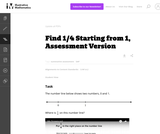Unrestricted Use
CC BY
Rating

This is a task from the Illustrative Mathematics website that is one part of a complete illustration of the standard to which it is aligned. Each task has at least one solution and some commentary that addresses important asects of the task and its potential use. Here are the first few lines of the commentary for this task: The number line below shows two numbers, 0 and 1. Where is $\frac14$ on this number line? http://www.youtube.com/watch?v=HJ0LDgxVGfU...

Subject:
Elementary Mathematics
Material Type:
Activity/Lab
Provider:
Illustrative Mathematics
Provider Set:
Illustrative Mathematics
Author:
Illustrative Mathematics
03/18/2013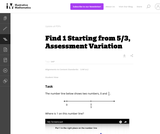Unrestricted Use
CC BY
Rating

This is a task from the Illustrative Mathematics website that is one part of a complete illustration of the standard to which it is aligned. Each task has at least one solution and some commentary that addresses important asects of the task and its potential use. Here are the first few lines of the commentary for this task: The number line below shows two numbers, 0 and $\frac53$. Where is 1 on this number line? http://www.youtube.com/watch?v=wAXRjMUgiu4...

Subject:
Elementary Mathematics
Material Type:
Activity/Lab
Provider:
Illustrative Mathematics
Provider Set:
Illustrative Mathematics
Author:
Illustrative Mathematics
03/18/2013Unrestricted Use
CC BY
Rating

This is a task from the Illustrative Mathematics website that is one part of a complete illustration of the standard to which it is aligned. Each task has at least one solution and some commentary that addresses important asects of the task and its potential use. Here are the first few lines of the commentary for this task: The number line below shows two numbers, 0 and 1. Where is $\frac74$ on this number line? http://www.youtube.com/watch?v=TEzH_PbHZIw...

Subject:
Elementary Mathematics
Material Type:
Activity/Lab
Provider:
Illustrative Mathematics
Provider Set:
Illustrative Mathematics
Author:
Illustrative Mathematics